Appendix A: The governing equations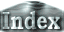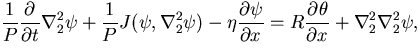(1)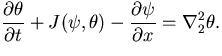(2)

The governing equations are non-dimensionalized using the thickness of layer d as length scale, thermal diffusion time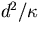as time scale,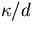as velocity scale, and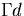as temperature scale.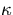is the thermal diffusivity and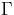is the absolute value of temperature gradient of the basic state determined by the heat flux at the boundaries.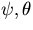are stream function and temperature disturbance, respectively.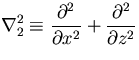is the two-dimensional Laplacian operator, and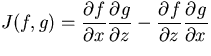is the Jacobian. Non-dimensional numbers appearing in the system are the Rayleigh number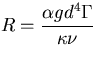, the Prandtl number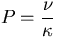, and the parameter of topographic b effect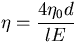, where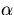is the thermal expansion coefficient, g is the gravitational acceleration,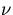is the kinematic viscosity,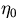is inclination of the boundaries, l is the size in the direction of the rotation axis, and E is the Ekman number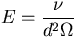.

 Appendix A: The governing equations